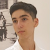# C# Types [Value, Reference] Explained

In C# we can find three different kinds of types, Value, Reference, Pointer types. In this article, we are going to focus on Reference & Value types.

So What are Reference & Value types?

# The Main Difference

The main difference between value & reference types is by their assignment.

`int a = 5;int b = a;b++;`

In the example above, we assign to the variable “b” the value of the variable “a”, and after that, we increase the value of b.

What is the value of b and what would be the value of a? Well, as you probably knew that…

# Intermediate C# [OOP & Structs, delegates]

## In C#, we can find some useful OOP tools to make our code better.

NOTE — The operations (operator overloading, indexers) would work for structs as well. However, the examples would use classes.

# Indexers

If you have ever used List<T> or Dictionary<T> and you were curious about how can an instance of a class can have indices just like an array, then the answer for this is indexers.

In the example below, we can see how to create an indexer.

T — Stands for the value we return from the index.

[int index] — The index the user gave…

# C# Tricks & Features

## Any programming language has its tricks and purposes.

Understanding the tricks behind any programming language can make your code to be shorter and more efficient.

# Expression-bodied members in Classes

In C# OOP we can find some useful tools that can save us a lot of time and place while writing code.

`public class SampleClass{    public int x { get; set; }    public int y { get; set; }    public int z => x + y; //automaticlly holds the sum}`

In the following example, we can see two basic declarations of variables.

The declaration of the x & y variable means that we can get & set their values from anywhere.